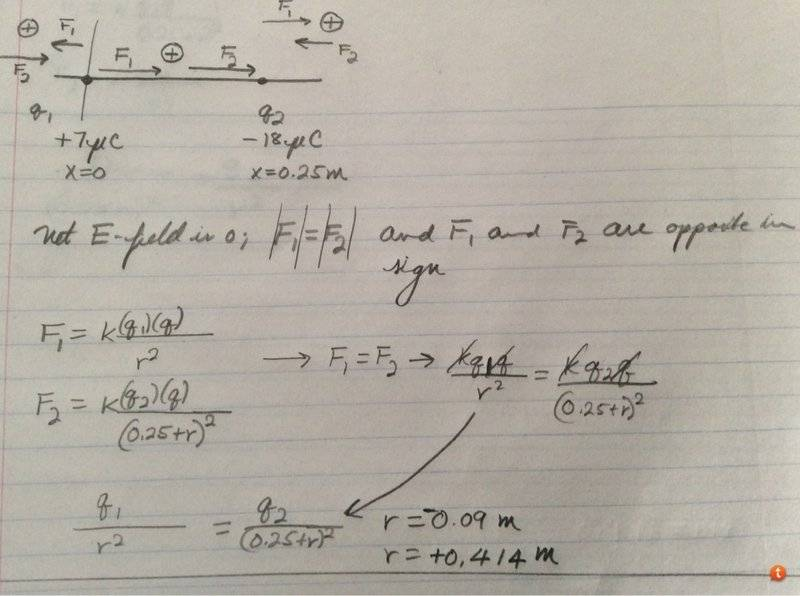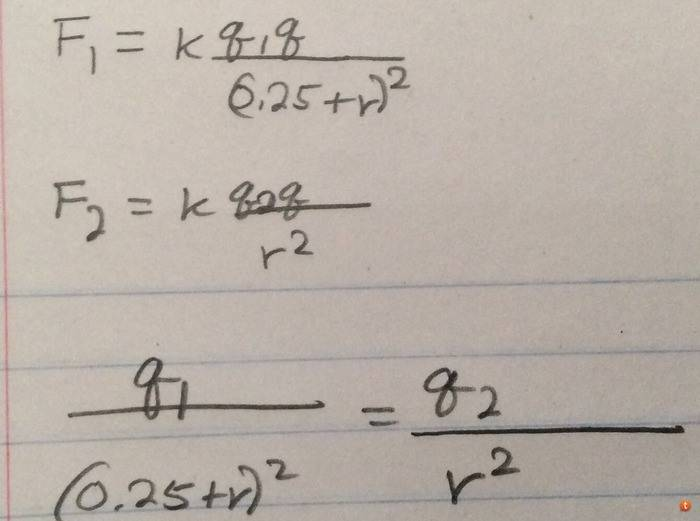# Electric field strength goes to 0

Gold Member

## Homework Statement

Two point charges lie on the x-axis. Charge Q1= +7 μC is located at x = 0 and charge Q2 = -18μC is located at x = 25 cm. Find the point on the x axis (in cm) where the net electric field is zero.

## Homework Equations

E = F/C

F = k(q1)(q2)/r^2

## The Attempt at a Solution

Okay, so I first did some common sense considerations. If the positive test charge particle lay in-between the q1 and q2, there is no way that via the principle of superposition the forces would cancel out (i.e. add destructively). The force on the test particle by q1 would be in the positive x direction (i.e. repulsion). Similarly, the force between the test particle by q2 would be in the positive x direction (in this case attraction creates a positive x-direction force on the test charge).

So the particle cannot be in-between q1 and q2. The particle either lies to the right of q1 and q2 or to the left of q1 and q2. If the particle lay right of q2, then F1 would be positive while F2 would be negative. If the particle lay left of q1, then F1 would be negative while F2 would be positive. Diagram attached.

So, what I am getting at is that there are at least two ways of destructively superimposing the two forces. Am I right?

Also, when I do the math, I don't seem to get quite the right answer. Let's say the particle were to the left of q1; math below:

I solve this system of equations and I get both a negative and a positive root. What does the negative root in this case mean? Go left on the x-axis?

http://www.wolframalpha.com/input/?i=7(0.25+r)^2+=+18r^2

Also if I solve this by assuming the particle is to the right of q2 ... I get these two values. Are these values valid?

http://www.wolframalpha.com/input/?i=7r^2+=+18(0.25+r)^2You are correct in stating that it is impossible for the electric force to cancel between the two particles. You are also correct that the fields will oppose each other to the left and to the right. Notice however you have charges of differing magnitudes. Electric field varies as the square of the distance, so looking at the magnitude of your charges, it will be impossible for the field to cancel in one of those regions. Your math looks correct, but if you multiply through,

$$\frac{q_1}{r^2} = \frac{q_2}{(r + l)^2}$$

Where for simplicity I have called the 0.25m l, then you have

$$q_1(r + l)^2 = rq_2$$

Use this method to try to see if you can get it down to one definite answer instead of two roots.

Last edited: